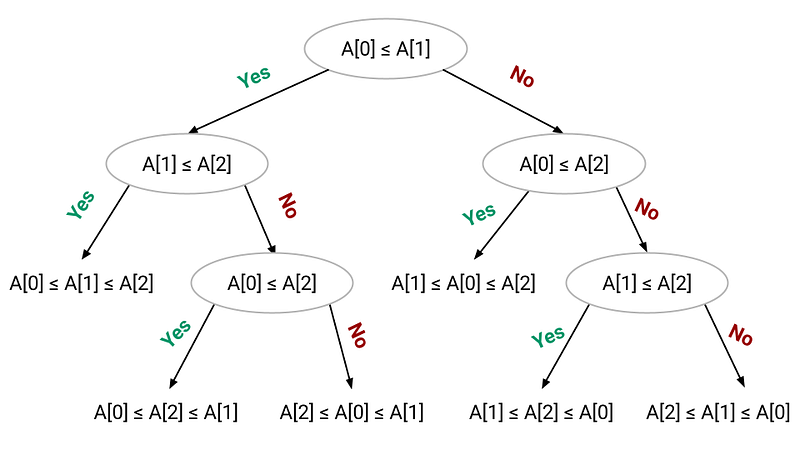# Lower bound of Comparison based Sorting

We have already discussed several sorting algorithms that can sort n numbers in both O(n²) and O(nlogn) time complexity in the worst case. These algorithms determine the sorted order based only on the comparisons between the input elements. We call such sorting algorithms comparison sort, where we use only comparisons between elements to gain order information about an input sequence A, A, . . . , A[n-1]. In other words, given two elements A[i] and A[j], we perform one of the tests A[i] <= A[j], A[i] >= A[j], A[i] = A[j] to determine their relative order. Here we assume without loss of generality that all of the input elements are distinct.

### Decision tree model of comparison sorting

We can visualize comparison sorts in terms of decision trees. A decision tree is a full binary tree structure that represents the comparisons performed by a sorting algorithm when it operates on an input of a given size. Here we only consider comparison operation and ignore other operations like control, data movement, etc.

Here is an example of a decision tree model for insertion sort operating on three elements. There are 3! = 6 possible permutations of the input elements, so the decision tree must have at least 6 leaves. Think!• Each internal node in the decision tree is represented by a comparison between a pair of elements (A[i], A[j]) for some i and j in the range 0 ≤ i, j ≤ n-1. if A[i] ≤ A[j] is true, we follow the left path, otherwise, we follow the right path.
• Each leaf node represents a permutation of the given input. In other words, each of the n! permutations on n elements must appear as one of the leaves of the decision tree for the sorting algorithm to sort properly.
• The execution of the sorting algorithm corresponds to tracing a path from the root of the decision tree to a leaf. At each internal node, a comparison A[i] ≤ A[j] is made. The left subtree then dictates subsequent comparisons for A[i] ≤ A[j] and the right subtree dictates subsequent comparisons for A[i]>A[j]. When we come to a leaf, the sorting algorithm has established the sorted ordering of the input elements.
• The height of the decision tree (length of the longest path from the root to any of its leaves) represents the worst-case number of comparisons the sorting algorithm performs. A lower bound on the heights of decision trees is therefore a lower bound on the running time of any comparison sort algorithm.

Idea: Any decision tree that sorts n elements has a height always greater than nlogn.

Proof : Consider a decision tree of height h that sorts n elements. Since there are n! permutations of n elements, each permutation representing a distinct sorted order, the tree must have at least n! leaves. Another side, we know that the binary tree of height h has no more than 2^h leaves.

``````=> n! ≤ 2^h
=> h ≥ log(n!)
From Stirling’s formula, n! > (n/e)^n
=> h ≥ log(n/e)^n
=> h ≥ n(log n — log e)
=> h ≥ nlogn — nloge
=> h > O(nlogn)
``````

Therefore, any comparison-based sorting algorithm must make at least O(nlogn) comparisons to sort the input array.

### Critical Ideas to think!

• Heapsort and merge sort are optimal comparison sorts.
• What is the smallest possible depth of a leaf in a decision tree?
• Prove that 2n-1 comparisons are necessary in the worst case to merge two sorted arrays containing n elements each.

### Introduction to linear time sorting

There are sorting algorithms that run faster than O(nlogn) time complexity but they require special assumptions about the input sequence. These sorting algorithms use operations other than comparisons to determine the sorted order and work in O(n) time complexity.

Examples of sorting algorithms that run in linear time are counting sort, radix sort, bucket sort, etc. Counting sort and radix sort assume that the input consists of integers in a small range. Whereas, bucket sort assumes that the input is generated by a random process that distributes elements uniformly over the interval.

### Suggested concepts and problems to explore

Reference: Algorithms by CLRS

Enjoy learning, Enjoy thinking!

Subscribe to get well-designed content on data structures and algorithms, machine learning, system design, oops, and mathematics. enjoy learning!

### We Welcome Doubts and Feedback!